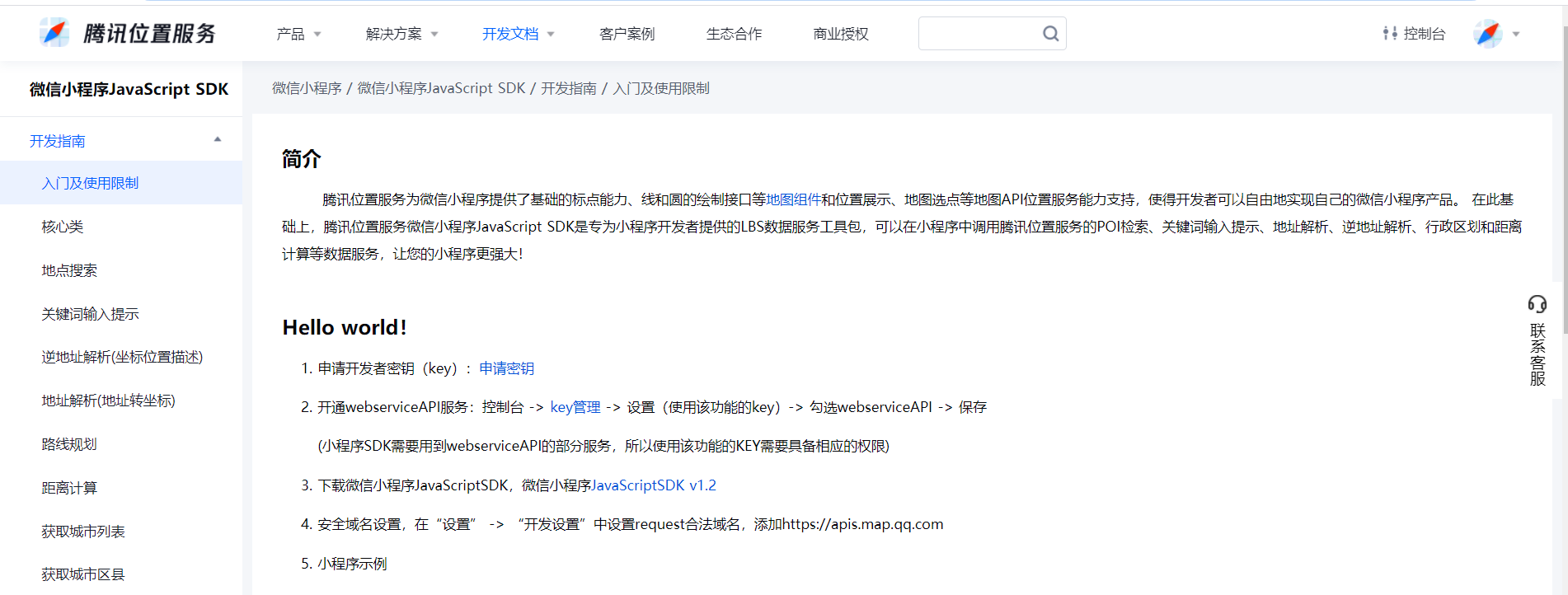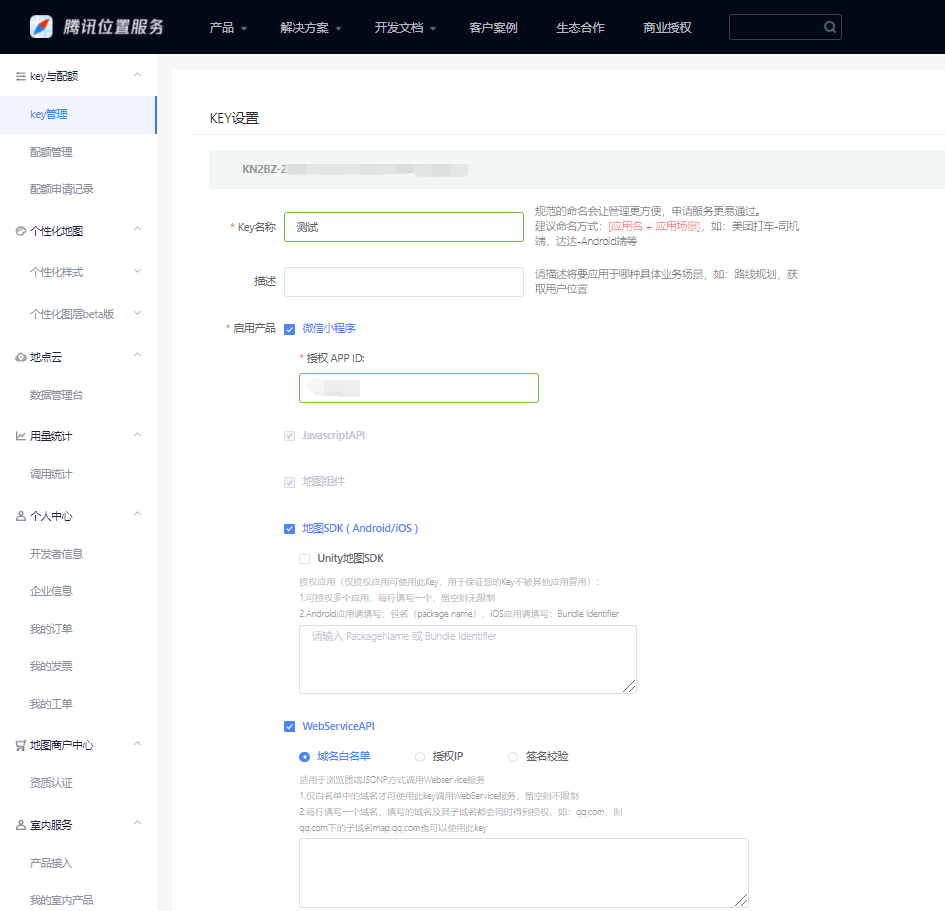# 置顶3分钟搞定微信小程序类美团用户商家距离计算精选热门

## 思路

1.利用小程序的wx.getLocation 方法得到用户的经纬度,然后用已知的商家的经纬进行计算;

2.利用腾讯地图位置服务calculateDistance直接计算；

longitude:经度;

latitude:纬度;

## 一、获取用户的位置信息,再进行计算(wx.getLocation)

1.小程序提供了获取用户位置信息的api，所以我们能直接获取到经纬度;

2.在腾讯位置服务坐标拾取器，获取商家的具体经纬度(例：北京故宫博物院116.397027(经度),39.917990(纬度))；

3.利用公式进行两点的经纬度计算，需注意：小程序默认坐标系是wgs84，您需设置为gcj02再试；

``````Page({
data:{
},
var _this = this;
_this.findXy() //查询用户与商家的距离
},

findXy() { //获取用户的经纬度
var _this = this
wx.getLocation({
type: 'gcj02',
success(res) {
_this.getDistance(res.latitude, res.longitude, 39.917990,116.397027)
}
})
},

return d * Math.PI / 180.0;
},
getDistance: function(lat1, lng1, lat2, lng2) {
// lat1用户的纬度
// lng1用户的经度
// lat2商家的纬度
// lng2商家的经度
var s = 2 * Math.asin(Math.sqrt(Math.pow(Math.sin(a / 2), 2) + Math.cos(radLat1) * Math.cos(radLat2) * Math.pow(Math.sin(b / 2), 2)));
s = s * 6378.137;
s = Math.round(s * 10000) / 10000;
s = s.toFixed(2) + '公里' //保留两位小数
console.log('经纬度计算的距离:' + s)
return s
}
)}
``````

## 二、利用腾讯地图的位置服务

1、先到腾讯位置服务注册登录，申请key、引入依赖。2、配置完成了之后，小程序重新编译一下

3、求两点距离文档：腾讯位置-两点求距

4、读完可知,我们只需要商家的经纬度即可,我们在小程序里实验一下

``````// 引入SDK核心类
var QQMapWX = require('../../utils/qqmap-wx-jssdk.js');

Page({
var _this = this;
_this.findShop() //查询用户与商家的距离
},
findShop() { //拿到商家的地理位置，用到了腾讯地图的api
// 实例化API核心类
var _that = this
var demo = new QQMapWX({
key: '你申请到的key' // 必填
});
// 调用接口
demo.calculateDistance({
to: [{

latitude: 39.917990, //商家的纬度
longitude: 116.397027, //商家的经度
}],
success: function(res) {
let hw = res.result.elements.distance //拿到距离(米)
if (hw && hw !== -1) { //拿到正确的值
//转换成公里
hw = (hw / 2 / 500).toFixed(2) + '公里'
} else {
hw = "距离太近或请刷新重试"
}

console.log('腾讯地图计算距离商家' + hw);
}
});
}
})
``````

{status:199,message:‘此key未开启webservice功能’}